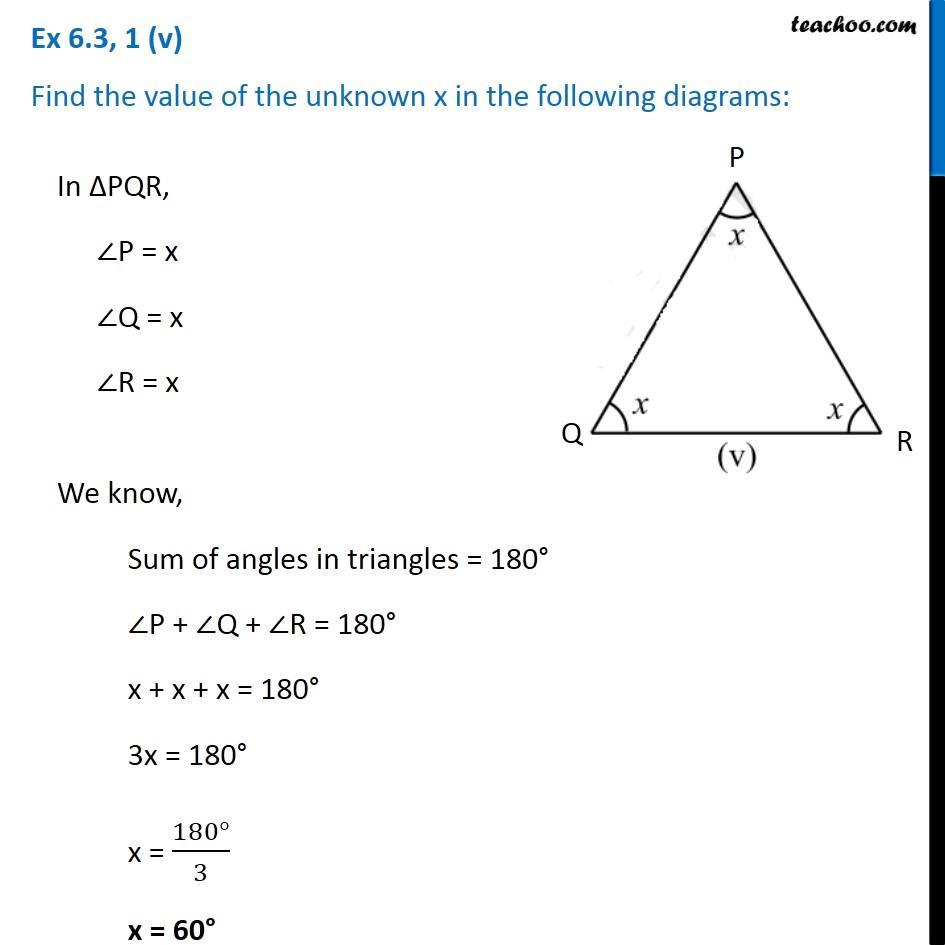Ex 6.3

Chapter 6 Class 7 Triangle and its Properties
Serial order wiseLearn in your speed, with individual attention - Teachoo Maths 1-on-1 Class

### Transcript

Ex 6.3, 1 (v) Find the value of the unknown x in the following diagrams:In ∆PQR, ∠P = x ∠Q = x ∠R = x We know, Sum of angles in triangles = 180° ∠P + ∠Q + ∠R = 180° x + x + x = 180° 3x = 180° x = (180°)/3 x = 60°